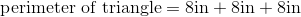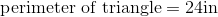# ISEE Lower Level Math : How to find the perimeter of a triangle

## Example Questions

### Example Question #21 : How To Find The Perimeter Of A Triangle

An isosceles triangle measuresinches along its two equal sides, andinches along its third side.  What is the perimeter of the triangle?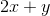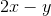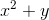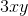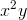Explanation:

To find the perimeter of any object, simply add the length of each side together.  The best answer is: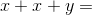### Example Question #22 : How To Find The Perimeter Of A Triangle

An equilateral triangle has a base of.  What is the perimeter?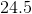Cannot be determined since you are given one side length only.Explanation:

An equilateral triangle has three equal sides so the side lengths of this triangle are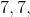and.

The perimeter is the total of the three sides so the answer is,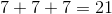.

### Example Question #361 : Geometry

Find the perimeter of an equilateral triangle that has a side with a length of 9cm.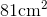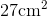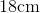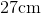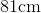Explanation:

The formula to find the perimeter of a triangle is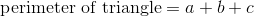where a, b, and c are the lengths of the sides.  When looking at equilateral triangles, we know that all lengths are equal.  Therefore, we know that each side is equal to 9cm.  So,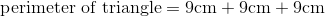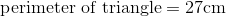### Example Question #362 : Geometry

Find the perimeter of the following triangle: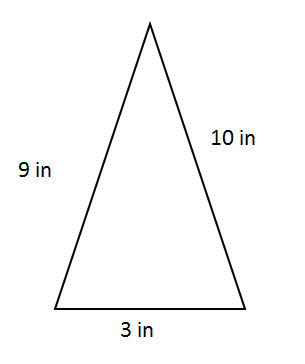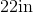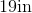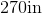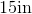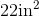Explanation:

To find the perimeter of a triangle, we will use the following formula:where a, b, and c are the lengths of each side of the triangle.

The sides on this triangle are of length 9in, 10in, and 3in.  So, we will add those lengths together.  We get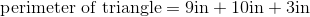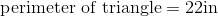### Example Question #363 : Geometry

An isosceles triangle measuresinches along its two equal sides, andinches along its third side.  What is the perimeter of the triangle?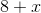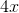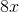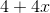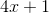Explanation:

To find the perimeter of any object, simply add the length of each side together.  The best answer is: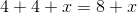### Example Question #364 : Geometry

An isosceles triangle measuresinches along its two equal sides, andinches along its third side.  What is the perimeter of the triangle?Explanation:

To find the perimeter of any object, simply add the length of each side together.  The best answer is: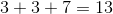### Example Question #365 : Geometry

An equilateral triangle measures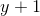inches along its three equal sides.  What is the perimeter of the triangle?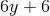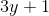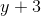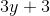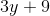Explanation:

To find the perimeter of any object, simply add the length of each side together.  The best answer is: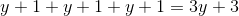### Example Question #23 : How To Find The Perimeter Of A Triangle

An equilateral triangle measures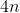inches along its three equal sides.  What is the perimeter of the triangle?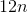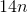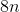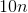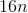Explanation:

To find the perimeter of any object, simply add the length of each side together.  The best answer is: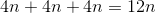### Example Question #367 : Geometry

Find the perimeter of an equilateral triangle with a side of length 13cm.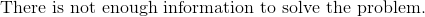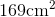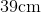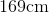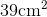Explanation:

To find the perimeter of a triangle, we use the following formula:where a, b, and c are the lengths of the sides of the triangle.

So, given the triangle mentioned above, we know that it is an equilateral triangle.  Because it is an equilateral triangle, we know that all the sides are equal.  The problem says one side is 13cm.  Therefore all sides are 13cm.  Knowing that, we can substitute into the formula.  We get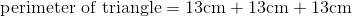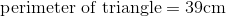### Example Question #368 : Geometry

Find the perimeter of an equilateral triangle with a base of 8 inches.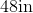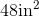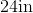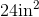Explanation:

To find the perimeter of a triangle, we use the following formula:where a, b, and c are the lengths of the sides of the triangle.

We know the length of the base of the triangle is 8 inches.  Because it is an equilateral triangle, we also know that all sides are equal.  Therefore, all sides are 8 inches.  Knowing this, we can substitute into the formula.  We get